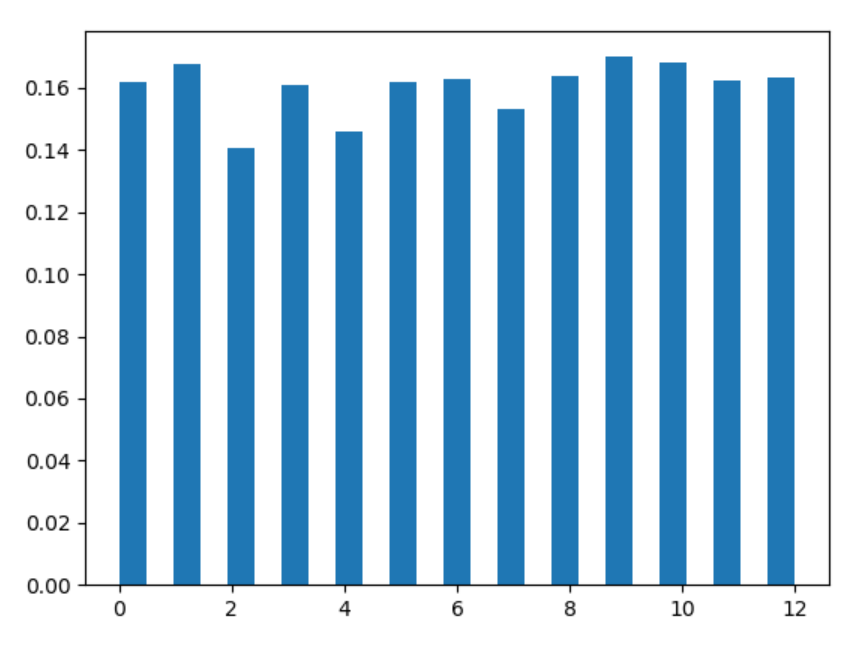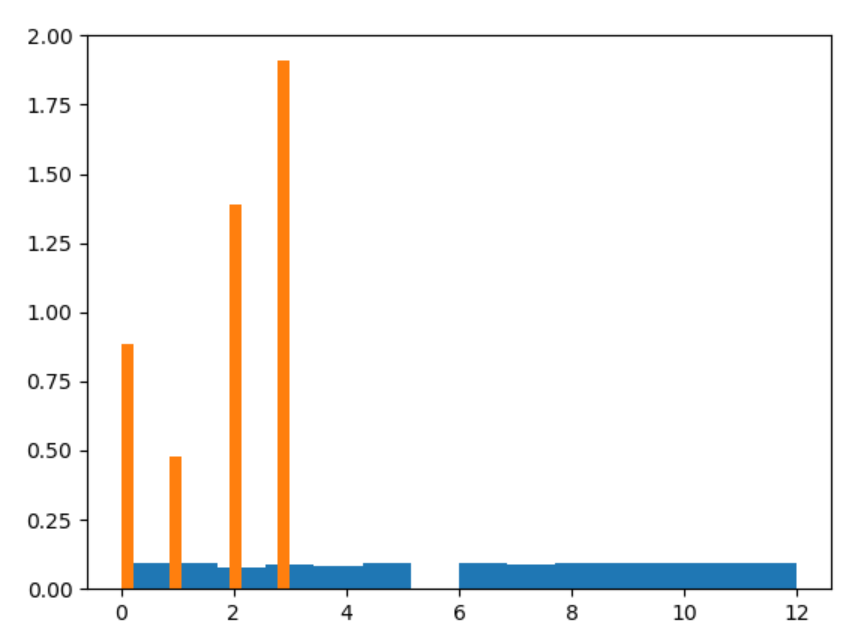Related Articles

# numpy.random.choice() in Python

• Difficulty Level : Expert
• Last Updated : 15 Jul, 2020

With the help of choice() method, we can get the random samples of one dimensional array and return the random samples of numpy array.

Syntax : numpy.random.choice(a, size=None, replace=True, p=None)

Attention geek! Strengthen your foundations with the Python Programming Foundation Course and learn the basics.

To begin with, your interview preparations Enhance your Data Structures concepts with the Python DS Course. And to begin with your Machine Learning Journey, join the Machine Learning - Basic Level Course

Parameters:

1) a – 1-D array of numpy having random samples.

2) size – Output shape of random samples of numpy array.

3) replace – Whether the sample is with or without replacement.

4) p – The probability attach with every samples in a.

Output : Return the numpy array of random samples.

Example #1 :

In this example we can see that by using choice() method, we are able to get the random samples of numpy array, it can generate uniform or non-uniform samples by using this method.

## Python3

 `# import choice``import` `numpy as np``import` `matplotlib.pyplot as plt`` ` `# Using choice() method``gfg ``=` `np.random.choice(``13``, ``5000``)`` ` `count, bins, ignored ``=` `plt.hist(gfg, ``25``, density ``=` `True``)``plt.show()`

Output :Example #2 :

## Python3

 `# import choice``import` `numpy as np``import` `matplotlib.pyplot as plt`` ` `# Using choice() method``gfg ``=` `np.random.choice(``5``, ``1000``, p ``=``[``0.2``, ``0.1``, ``0.3``, ``0.4``, ``0``])`` ` `count, bins, ignored ``=` `plt.hist(gfg, ``14``, density ``=` `True``)``plt.show()`

Output :My Personal Notes arrow_drop_up